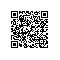# 1. 马尔科夫链的细致平稳条件

在解决从平稳分布ππ, 找到对应的马尔科夫链状态转移矩阵PP之前，我们还需要先看看马尔科夫链的细致平稳条件。定义如下：

如果非周期马尔科夫链的状态转移矩阵PP和概率分布π(x)π(x)对于所有的i,ji,j满足：

π(i)P(i,j)=π(j)P(j,i)π(i)P(i,j)=π(j)P(j,i)

则称概率分布π(x)π(x)是状态转移矩阵PP的平稳分布。

证明很简单,由细致平稳条件有：

i=1π(i)P(i,j)=i=1π(j)P(j,i)=π(j)i=1P(j,i)=π(j)∑i=1∞π(i)P(i,j)=∑i=1∞π(j)P(j,i)=π(j)∑i=1∞P(j,i)=π(j)

将上式用矩阵表示即为：

πP=ππP=π

即满足马尔可夫链的收敛性质。也就是说，只要我们找到了可以使概率分布π(x)π(x)满足细致平稳分布的矩阵PP即可。这给了我们寻找从平稳分布ππ, 找到对应的马尔科夫链状态转移矩阵PP的新思路。

不过不幸的是，仅仅从细致平稳条件还是很难找到合适的矩阵PP。比如我们的目标平稳分布是π(x)π(x),随机找一个马尔科夫链状态转移矩阵QQ,它是很难满足细致平稳条件的，即：

π(i)Q(i,j)π(j)Q(j,i)π(i)Q(i,j)≠π(j)Q(j,i)

那么如何使这个等式满足呢？下面我们来看MCMC采样如何解决这个问题。

# 2. MCMC采样

由于一般情况下，目标平稳分布π(x)π(x)和某一个马尔科夫链状态转移矩阵QQ不满足细致平稳条件，即

π(i)Q(i,j)π(j)Q(j,i)π(i)Q(i,j)≠π(j)Q(j,i)

我们可以对上式做一个改造，使细致平稳条件成立。方法是引入一个α(i,j)α(i,j),使上式可以取等号，即：

π(i)Q(i,j)α(i,j)=π(j)Q(j,i)α(j,i)π(i)Q(i,j)α(i,j)=π(j)Q(j,i)α(j,i)

问题是什么样的α(i,j)α(i,j)可以使等式成立呢？其实很简单，只要满足下两式即可：

α(i,j)=π(j)Q(j,i)α(i,j)=π(j)Q(j,i)
α(j,i)=π(i)Q(i,j)α(j,i)=π(i)Q(i,j)

这样，我们就得到了我们的分布π(x)π(x)对应的马尔科夫链状态转移矩阵PP，满足：

P(i,j)=Q(i,j)α(i,j)P(i,j)=Q(i,j)α(i,j)

也就是说，我们的目标矩阵PP可以通过任意一个马尔科夫链状态转移矩阵QQ乘以α(i,j)α(i,j)得到。α(i,j)α(i,j)我们有一般称之为接受率。取值在[0,1]之间，可以理解为一个概率值。即目标矩阵PP可以通过任意一个马尔科夫链状态转移矩阵QQ以一定的接受率获得。这个很像我们在MCMC(一)蒙特卡罗方法第4节讲到的接受-拒绝采样，那里是以一个常用分布通过一定的接受-拒绝概率得到一个非常见分布，这里是以一个常见的马尔科夫链状态转移矩阵QQ通过一定的接受-拒绝概率得到目标转移矩阵PP,两者的解决问题思路是类似的。

好了，现在我们来总结下MCMC的采样过程。

1）输入我们任意选定的马尔科夫链状态转移矩阵QQ，平稳分布π(x)π(x)，设定状态转移次数阈值n1n1，需要的样本个数n2n2

2）从任意简单概率分布采样得到初始状态值x0x0

3）for t=0t=0 to n1+n21n1+n2−1

a) 从条件概率分布Q(x|xt)Q(x|xt)中采样得到样本xx∗

b) 从均匀分布采样uuniform[0,1]u∼uniform[0,1]

c) 如果u<α(xt,x)=π(x)Q(x,xt)u<α(xt,x∗)=π(x∗)Q(x∗,xt), 则接受转移xtxxt→x∗，即xt+1=xxt+1=x∗

d) 否则不接受转移，t=max(t1,0)t=max(t−1,0)

样本集(xn1,xn1+1,...,xn1+n21)(xn1,xn1+1,...,xn1+n2−1)即为我们需要的平稳分布对应的样本集。

上面这个过程基本上就是MCMC采样的完整采样理论了，但是这个采样算法还是比较难在实际中应用，为什么呢？问题在上面第三步的c步骤，接受率这儿。由于α(xt,x)α(xt,x∗)可能非常的小，比如0.1，导致我们大部分的采样值都被拒绝转移，采样效率很低。有可能我们采样了上百万次马尔可夫链还没有收敛，也就是上面这个n1n1要非常非常的大，这让人难以接受，怎么办呢？这时就轮到我们的M-H采样出场了。

# 3. M-H采样

M-H采样是Metropolis-Hastings采样的简称，这个算法首先由Metropolis提出，被Hastings改进，因此被称之为Metropolis-Hastings采样或M-H采样

M-H采样解决了我们上一节MCMC采样接受率过低的问题。

我们回到MCMC采样的细致平稳条件：

π(i)Q(i,j)α(i,j)=π(j)Q(j,i)α(j,i)π(i)Q(i,j)α(i,j)=π(j)Q(j,i)α(j,i)

我们采样效率低的原因是α(i,j)α(i,j)太小了，比如为0.1，而α(j,i)α(j,i)为0.2。即：

π(i)Q(i,j)×0.1=π(j)Q(j,i)×0.2π(i)Q(i,j)×0.1=π(j)Q(j,i)×0.2

这时我们可以看到，如果两边同时扩大五倍，接受率提高到了0.5，但是细致平稳条件却仍然是满足的，即：

π(i)Q(i,j)×0.5=π(j)Q(j,i)×1π(i)Q(i,j)×0.5=π(j)Q(j,i)×1

这样我们的接受率可以做如下改进，即：

α(i,j)=min{π(j)Q(j,i)π(i)Q(i,j),1}α(i,j)=min{π(j)Q(j,i)π(i)Q(i,j),1}

通过这个微小的改造，我们就得到了可以在实际应用中使用的M-H采样算法过程如下：

1）输入我们任意选定的马尔科夫链状态转移矩阵QQ，平稳分布π(x)π(x)，设定状态转移次数阈值n1n1，需要的样本个数n2n2

2）从任意简单概率分布采样得到初始状态值x0x0

3）for t=0t=0 to n1+n21n1+n2−1

a) 从条件概率分布Q(x|xt)Q(x|xt)中采样得到样本xx∗

b) 从均匀分布采样uuniform[0,1]u∼uniform[0,1]

c) 如果u<α(xt,x)=min{π(j)Q(j,i)π(i)Q(i,j),1}u<α(xt,x∗)=min{π(j)Q(j,i)π(i)Q(i,j),1}, 则接受转移xtxxt→x∗，即xt+1=xxt+1=x∗

d) 否则不接受转移，t=max(t1,0)t=max(t−1,0)

样本集(xn1,xn1+1,...,xn1+n21)(xn1,xn1+1,...,xn1+n2−1)即为我们需要的平稳分布对应的样本集。

很多时候，我们选择的马尔科夫链状态转移矩阵QQ如果是对称的，即满足Q(i,j)=Q(j,i)Q(i,j)=Q(j,i),这时我们的接受率可以进一步简化为：

α(i,j)=min{π(j)π(i),1}α(i,j)=min{π(j)π(i),1}

# 4. M-H采样实例

为了更容易理解，这里给出一个M-H采样的实例。

在例子里，我们的目标平稳分布是一个均值3，标准差2的正态分布，而选择的马尔可夫链状态转移矩阵Q(i,j)Q(i,j)的条件转移概率是以ii为均值,方差1的正态分布在位置jj的值。这个例子仅仅用来让大家加深对M-H采样过程的理解。毕竟一个普通的一维正态分布用不着去用M-H采样来获得样本。

代码如下：

import random
import math
from scipy.stats import norm
import matplotlib.pyplot as plt
%matplotlib inline

def norm_dist_prob(theta):
y = norm.pdf(theta, loc=3, scale=2)
return y

T = 5000
pi = [0 for i in range(T)]
sigma = 1
t = 0
while t < T-1:
t = t + 1
pi_star = norm.rvs(loc=pi[t - 1], scale=sigma, size=1, random_state=None)
alpha = min(1, (norm_dist_prob(pi_star) / norm_dist_prob(pi[t - 1])))

u = random.uniform(0, 1)
if u < alpha:
pi[t] = pi_star
else:
pi[t] = pi[t - 1]

plt.scatter(pi, norm.pdf(pi, loc=3, scale=2))
num_bins = 50
plt.hist(pi, num_bins, normed=1, facecolor='red', alpha=0.7)
plt.show()

输出的图中可以看到采样值的分布与真实的分布之间的关系如下，采样集还是比较拟合对应分布的。# 5. M-H采样总结

M-H采样完整解决了使用蒙特卡罗方法需要的任意概率分布样本集的问题，因此在实际生产环境得到了广泛的应用。

但是在大数据时代，M-H采样面临着两大难题：

1） 我们的数据特征非常的多，M-H采样由于接受率计算式π(j)Q(j,i)π(i)Q(i,j)π(j)Q(j,i)π(i)Q(i,j)的存在，在高维时需要的计算时间非常的可观，算法效率很低。同时α(i,j)α(i,j)一般小于1，有时候辛苦计算出来却被拒绝了。能不能做到不拒绝转移呢？

2） 由于特征维度大，很多时候我们甚至很难求出目标的各特征维度联合分布，但是可以方便求出各个特征之间的条件概率分布。这时候我们能不能只有各维度之间条件概率分布的情况下方便的采样呢？

Gibbs采样解决了上面两个问题，因此在大数据时代，MCMC采样基本是Gibbs采样的天下，下一篇我们就来讨论Gibbs采样。使用钉钉扫一扫加入圈子
+ 订阅